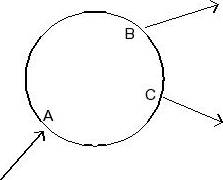# Horizontal ring water main pressure drop

## Homework Statement

The accompanying figure shows a horizontal ring main consisting of a single loop of pipe, as might be used for supplying water to various points on one floor of a building. Water enters the main at A at 50 psig and is discharged at B and C at rates of 20 gpm and 60 gpm, respectively. Tests on the particular pipe forming the main show that the frictional pressure drop
(psi) is given by –Δp = 0.0002Q2L, where L is the pipe length in feet and Q is the flow rate in gpm. Estimate the flow rates in the individual sections AB, BC and AC, and the pressures at B and C. The distances are AB = 100 ft, BC = 50 ft and AC = 200 ft## The Attempt at a Solution

I used the mechanical energy balance.
I'm left with P1/rho=(P2/rho)+hf
hf= Pipeline + Minor Losses
hf= Pipeline + 0
Pipeline= ((4fL)/D)*(Velocity^2)/2
mass flow= 80 gpm

BvU